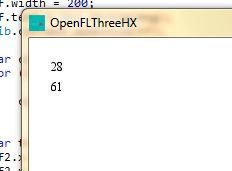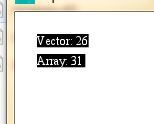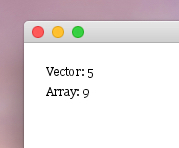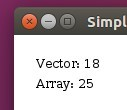# Optimize Performance from Haxe like AS3?

Hello everyone,

I check AS3 with Air captive and Haxe with OpenFL CPP

How does vector run fast?

AS3: Vector. = 47

Haxe Vector = 26

Stackoverflow

And Vector class versus Array class:

My code for Haxe example:
package;

``````import openfl.display.Sprite;
import openfl.text.TextField;
import openfl.Lib;
import openfl.Vector;

/**
* ...
* @author Jens Eckervogt
*/
class Main extends Sprite
{

public function new()
{
super();

var MAX_NUM:UInt = 300000;
var coordinates:Vector<Float> = new Vector<Float>(MAX_NUM, true);
var started:UInt = Lib.getTimer();

for (i in 0...MAX_NUM)
{
coordinates[i] = Math.random()*1024;
}

var msg:Int = Lib.getTimer() - started;

var tf:TextField = new TextField();
tf.x = tf.y = 20;
tf.width = 200;
tf.text = Std.string(msg);

}

}
``````

Output 26 - 28

Is it fast?

If you want create TileMap but I would like to suggest with `Vector<Int>` or `Vector<UInt>` if you have many tiles with biggest resolution

Thanks!

Using openfl.Vector does not make array access significantly faster because it’s internally backed by Array. It might be slightly slower because it uses virtual function calls for template specialization.

On Haxe Array is typed, and at least on cpp it’s backed by continuous memory.
On JavaScript it’s impossible to explicitly use fixed size arrays (except for typed arrays), but even with Array, VM may decide to use fixed arrays when possible.

I don’t use openfl.Vector unless I have a special reason to do so (e.g. when I need compatibility with Flash).

Only advantage of using openfl.Vector might be that you can set length in constructor.
Filling arrays with default value is waste of time on JavaScript though.

Why not?
I have tried Vector and Array:

Output: Vector 28, Array 61.Code:
package;

``````import openfl.display.Sprite;
import openfl.text.TextField;
import openfl.Lib;
import openfl.Vector;

/**
* ...
* @author Jens Eckervogt
*/
class Main extends Sprite
{

public function new()
{
super();

var MAX_NUM:UInt = 300000;
var coordinates:Vector<Float> = new Vector<Float>(MAX_NUM, true);
var started:UInt = Lib.getTimer();

for (i in 0...MAX_NUM)
{
coordinates[i] = Math.random()*1024;
}

var msg:Int = Lib.getTimer() - started;

var tf:TextField = new TextField();
tf.x = tf.y = 20;
tf.width = 200;
tf.text = Std.string(msg);

var coordinatesA:Array<Float> = [MAX_NUM];
for (i in 0...MAX_NUM)
{
coordinatesA[i] = Math.random()*1024;
}

var tf2:TextField = new TextField();
tf2.x = 20;
tf2.y = 40;
tf2.width = 200;
tf2.text = Std.string(Lib.getTimer() - started);
}

}
``````

Thanks!

Not a fair comparison… You are not initializing `started` in second test.
Also, you are creating an array that contains only one element in second test. `var coordinatesA:Array<Float> = [MAX_NUM];` If you create an array with very small size, it sometimes increases capacity to store all elements.

`openfl.Vector` is really backed by Arrays now, see source code.

You are creating 2 Textfields during your second test.
Note also that you should test on each target and test reading (not only writing).

I tried modifying and running your test with this code:

``````import openfl.display.Sprite;
import openfl.text.TextField;
import openfl.Lib;
import openfl.Vector;

/**
* ...
* @author Jens Eckervogt
*/
class Main extends Sprite
{

public function new()
{
super();

var MAX_NUM:UInt = 300000;
var coordinates:Vector<Float> = new Vector<Float>(MAX_NUM, true);
var started:UInt = Lib.getTimer();

for (i in 0...MAX_NUM)
{
coordinates[i] = Math.random()*1024;
}

var msg:Int = Lib.getTimer() - started;

var tf:TextField = new TextField();
tf.x = tf.y = 20;
tf.width = 200;
tf.text = Std.string("Vector: " + msg);

var coordinatesA:Array<Float> = [MAX_NUM];
started = Lib.getTimer ();

for (i in 0...MAX_NUM)
{
coordinatesA[i] = Math.random()*1024;
}

msg = Lib.getTimer() - started;

var tf2:TextField = new TextField();
tf2.x = 20;
tf2.y = 40;
tf2.width = 200;
tf2.text = Std.string("Array: " + msg);
}

}
``````

I got these results:

Vector: 16
Array: 46

Vector: 74
Array: 86

Vector: 22
Array: 27

### HTML5

Vector: 27
Array: 27

The results vary from run to run (especially on HTML5) but this is a rough average of results I saw

This said, `Vector` might make the most sense for Tilemap

Wow!!! Thanks for modifying …
My computer is very old but it is very faster why???

Flash:
Vector 9
Array: 36

C++/Header: ( All operating systems ):
Vector 28
Array: 36

neko::
Vector 158
Array 134

HTML5/JS:
Internet Explorer 11
-> Vector 20
-> Array 17
-> Vector 50
-> Array 17
Mozilla Firefox
-> Vector 7
-> Array 16
Opera 12.x Not tested …
Netgear ( very old browser ) not tested…

Wow I am surprised because Mozilla Firefox is faster than all browsers because Fox loves only vector and fox hates arrays why does fox not like to love with arrays?

But other browsers love only arrays so that I don’t understand why do browsers like only arrays?

I can’t understand because Firefox hates arrays much if Firefox freezes large Tilemap

// EDIT:
My brother suggests me because somebody doesn’t want add more textfields just make trick like this
Example:
X = space_x + textwidth + space_x + textwidth
or Y = space_y + textheight + space_y + textheight
Just multiply with posX or posY
than it happens for example code.
I have changed into simple calculation like
if posY is 0 mean it stays current height of space 20 than textfield hangs from space of top stage edge.

I show you:

``````package;

import openfl.display.Sprite;
import openfl.text.TextField;
import openfl.Lib;
import openfl.Vector;

/**
* ...
* @author Jens Eckervogt
*/
class Main extends Sprite
{

public function new()
{
super();

var MAX_NUM:UInt = 300000;
var coordinates:Vector<Float> = new Vector<Float>(MAX_NUM, true);
var started:UInt = Lib.getTimer();

for (i in 0...MAX_NUM)
{
coordinates[i] = Math.random()*1024;
}

var msg:Int = Lib.getTimer() - started;

var coordinatesA:Array<Float> = [MAX_NUM];
started = Lib.getTimer ();

for (i in 0...MAX_NUM)
{
coordinatesA[i] = Math.random()*1024;
}

msg = Lib.getTimer() - started;

}

{
var textfield:TextField = new TextField();
textfield.text = s.toString();
textfield.x = 20;
textfield.y = 20 + (posY * 20);
textfield.width = 50 + textfield.text.length;
return textfield;
}
}
``````

Result: It doesn’t happen now.That is good and I hope you can make my idea if you add more textfield from
`private function addLogViewer(s:Dynamic, posX:Int, posY:Int):TextField` for example - If you don’t want get problem with HaxeUI via fast-functions

I will try for fast functions

HTML5 varies a lot in the results I saw, reload each browser a few times, and you’ll see the numbers jump around

You may need to run warmup to get stable results on non-cpp. Maybe test order need to be swapped to get more accurate resluts.

Why are you still using small array in second test??? Again that’s not fair. `［MAX_NUM］` just creates an array that contains 1 element, not MAX_NUM elments.

But as @singmajesty said result varies between each run… Would be better to test this on real-world applications.
I still don’t think Vector is faster on non-flash because it has more overhead.

Also we should test vector with non finite length.

The abstract Vector class in OpenFL is going to likely perform similar to Array, except that it can allocate a length in advance, so it will perform faster than a lot of `push` calls

Very correctly!

Example three js has Vector2 functions like toArray and fromArray i replace to toVector and fromVector and Vector has same properties and methods like to array class.

// EDIT I am back now because my new computer was upgraded.
Intel Processor i7 6700, ssd harddisk 750 gb, Display Driver AMD Radeon RX 480 8 GB VideoRam and 16 GB Ram.

Now I am using under VMWare Player and macOS Sierra works fine for me. Yay It is not hackintosh just real macintosh without virtualgl support - I know I want try to create new simple vmgl-like kext for macOS sierra 10.12.1

I am very happy because Haxe works fine under my mac os and it is 3.4.0 rc1 ( ps. old version doesn’t work 3.2.0 gets error…

I have checked vector and array wow so fast like my new computer has power…It is not very slow for me. I am very happy with macOS now.
and i am using result:

Sorry my picture is very big because i have new display monitor dell 27 inch…

Thanks!

Next test for Ubuntu/LinuxThanks!